# Review: Distributed Representations of Sentences and Documents (Doc2Vec)

## Paragraph Vectors Obtained Using PV-DM and PV-DBOW

In this story, Distributed Representations of Sentences and Documents, (Doc2Vec), by Google, is reviewed. In this paper:

• Paragraph vector is introduced, which is an unsupervised algorithm that learns fixed-length feature representations from variable-length pieces of texts, such as sentences, paragraphs, and documents.
• Each document is represented by a dense vector which is trained to predict words in the document.

This is a paper in 2014 ICML with over 8300 citations. (Sik-Ho Tsang @ Medium)

# Outline

1. Conventional Approach for Learning Vector Representation of Words
2. Distributed Memory Model of Paragraph Vectors (PV-DM)
3. Distributed Bag of Words version of Paragraph Vector (PV-DBOW)
4. Experimental Results

# 1. Conventional Approach for Learning Vector Representation of Words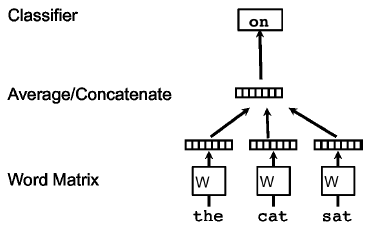Conventional Approach for Learning Vector Representation of Words
• In this framework, every word is mapped to a unique vector, represented by a column in a matrix W. The column is indexed by position of the word in the vocabulary.
• The concatenation or sum of the vectors is then used as features for prediction of the next word in a sentence.
• As shown above, context of three words (“the,” “cat,” and “sat”) is used to predict the fourth word (“on”). The input words are mapped to columns of the matrix W to predict the output word.
• More formally, given a sequence of training words w1, w2, w3, …, wT, the objective of the word vector model is to maximize the average log probability:
• The prediction task is typically done via a multiclass classifier, such as softmax:
• Each of yi is un-normalized log-probability for each output word i, computed as (eq.(1)):
• where U, b are the softmax parameters. h is constructed by a concatenation or average of word vectors extracted from W.
• (Not so important in the paper) Here, hierarchical softmax, suggested by Prof. Hinton, is used for fast training. the structure of the hierarchical softmax is a binary Huffman tree, where short codes are assigned to frequent words. This is a good speedup trick because common words are accessed quickly.

After the training converges, words with similar meaning are mapped to a similar position in the vector space. For example, “powerful” and “strong” are close to each other, whereas “powerful” and “Paris” are more distant.

The difference between word vectors also carry meaning. For example: “King”-“man”+“woman”=“Queen”.

• These properties make word vectors attractive for many natural language processing (NLP) tasks such as language modeling, natural language understanding, statistical machine translation, image understanding and relational extraction.

# 2. Distributed Memory Model of Paragraph Vectors (PV-DM)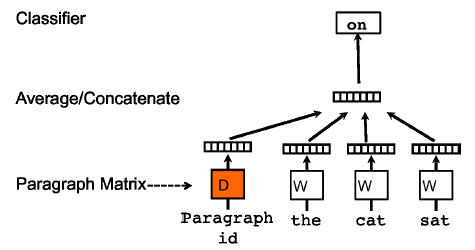Distributed Memory Model of Paragraph Vectors (PV-DM)
• In the proposed Paragraph Vector framework, every paragraph is mapped to a unique vector, represented by a column in matrix D and every word is also mapped to a unique vector, represented by a column in matrix W.
• The paragraph vector and word vectors are averaged or concatenated to predict the next word in a context. concatenation is used as the method to combine the vectors.
• More formally, the only change in this model compared to the word vector framework is in eq.(1), where h is constructed from W and D.
• The contexts are fixed-length and sampled from a sliding window over the paragraph. The paragraph vector is shared across all contexts generated from the same paragraph but not across paragraphs.
• The word vector matrix W, however, is shared across paragraphs.

An important advantage of paragraph vectors is that they are learned from unlabeled data and thus can work well for tasks that do not have enough labeled data.

# 3. Distributed Bag of Words version of Paragraph Vector (PV-DBOW)

## 3.1. PV-DBOW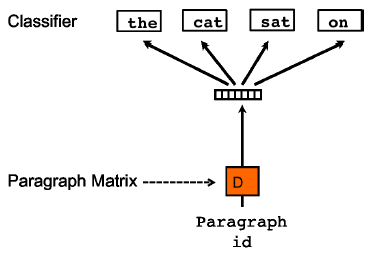Distributed Bag of Words version of Paragraph Vector (PV-DBOW)
• Another way is to ignore the context words in the input, but force the model to predict words randomly sampled from the paragraph in the output.
• This model requires to store less data. We only need to store the softmax weights.
• This model is also similar to the Skip-gram model in Word2Vec.

## 3.2. PV-DM + PV-DBOW

• In the experiments, each paragraph vector is a combination of two vectors: one learned by the standard paragraph vector with distributed memory (PV-DM) and one learned by the paragraph vector with distributed bag of words (PV-DBOW).

# 4. Experimental Results

## 4.1.1. Dataset

• This dataset has 11855 sentences taken from the movie review site Rotten Tomatoes. The dataset consists of three sets: 8544 sentences for training, 2210 sentences for test and 1101 sentences for validation.
• Every sentence in the dataset has a label which goes from very negative to very positive in the scale from 0.0 to 1.0. The labels are generated by human annotators using Amazon Mechanical Turk.
• There are two ways of benchmarking. First, one could consider a 5-way fine-grained classification task where the labels are {Very Negative, Negative, Neutral, Positive, Very Positive} or a 2-way coarse-grained classification task where the labels are {Negative, Positive}.

## 4.1.2. Training

• After learning the vector representations for training sentences and their subphrases, they are fed to a logistic regression to learn a predictor of the movie rating.
• At test time, the vector representation is frozen for each word, and to learn the representations for the sentences using gradient descent.
• Once the vector representations for the test sentences are learned, they are fed through the logistic regression to predict the movie rating.
• The optimal window size is 8, i.e. to predict the 8-th word, the paragraph vectors are concatenated with 7 word vectors.
• The vector presented to the classifier is a concatenation of two vectors, one from PV-DBOW and one from PV-DM, each with 400 dimensions.

## 4.1.3. Results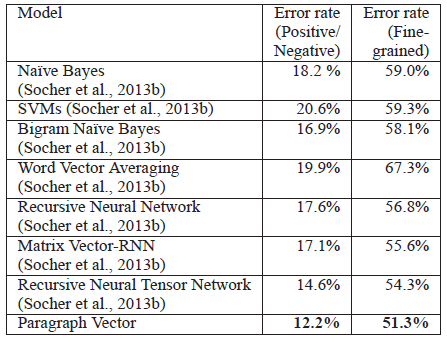The performance of our method compared to other approaches on the Stanford Sentiment Treebank dataset
• The first highlight for this Table is that bag-of-words or bag-of-n-grams models (NB, SVM, BiNB) perform poorly. Simply averaging the word vectors (in a bag-of-words fashion) does not improve the results. This is because bag-of-words models do not consider how each sentence is composed (e.g., word ordering) and therefore fail to recognize many sophisticated linguistic phenomena, for instance sarcasm.

The proposed method performs better than all the baselines.

## 4.2.1. Dataset

• The dataset consists of 100,000 movie reviews taken from IMDB. They are divided into three datasets: 25,000 labeled training instances, 25,000 labeled test instances and 50,000 unlabeled training instances. There are two types of labels: Positive and Negative.

## 4.2.2. Training

• The training is similar to the Stanford Sentiment Treebank Dataset one.
• The optimal window size is 10.
• The vector presented to the classifier is a concatenation of two vectors, one from PV-DBOW and one from PV-DM, each with 400 dimensions.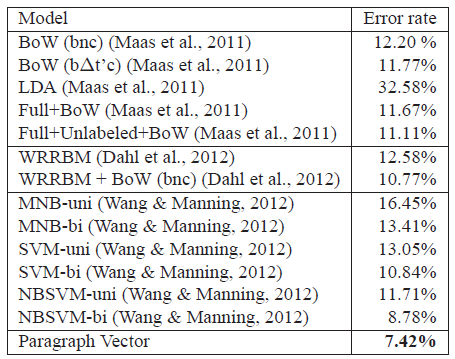The performance of Paragraph Vector compared to other approaches on the IMDB dataset
• The method described in this paper is the only approach that goes significantly beyond the barrier of 10% error rate.

It achieves 7.42% which is another 1.3% absolute improvement (or 15% relative improvement) over the best previous result.

## 4.3.1. Dataset

• Authors have a dataset of paragraphs in the first 10 results returned by a search engine given each of 1,000,000 most popular queries. Each of these paragraphs is also known as a “snippet” which summarizes the content of a web page.
• For each query, a triplet of paragraphs is created: the two paragraphs are results of the same query, whereas the third paragraph is a randomly sampled paragraph from the rest of the collection (returned as the result of a different query).
• The goal is to identify which of the three paragraphs are results of the same query. A better representation is one that achieves a small distance for pairs of paragraphs of the same query and a large distance for pairs of paragraphs of different queries.
• The triplets are split into three sets: 80% for training, 10% for validation, and 10% for testing.

## 4.3.2. Results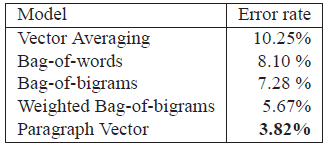The performance of Paragraph Vector and bag-of-words models on the information retrieval task

Paragraph Vector works well and gives a 32% relative improvement in terms of error rate.

## Reference

[2014 ICML] [Doc2Vec]
Distributed Representations of Sentences and Documents

## Natural Language Processing

Sequence Model: 2014 [GRU] [Doc2Vec]
Language Model: 2007 [Bengio TNN’07] 2013 [Word2Vec] [NCE] [Negative Sampling]
Machine Translation: 2014
[Seq2Seq] [RNN Encoder-Decoder] 2015 [Attention Decoder/RNNSearch]
Image Captioning: 2015 [m-RNN] [R-CNN+BRNN] [Show and Tell/NIC]

--

--